# Corrections to Multilevel Statistical Models - 3rd Edition

• P 33: line 5 up; 'of n-1'
• P 57: Insert + before uj
• P 86: 5 lines below eqn (3.22) delete 'back transform………and'
• P 86: 6 lines below eqn (3.22) change 'with the corresponding' to 'with correspondingly sampled'
• P 89: The 'star' in the diagram for line labelled 'C' should lie on that line 3mm along it below the solid line.
• P 112: 9 lines up change 'second line' to 'last two lines'. 14 lines up change 'second' to 'first'. 16 lines up change 'first' to 'third' and 'second' to 'first'
• P 123: 4 lines below eqn (4.3.3) change to
• P 131: line 2 above sect 5.4 'achievement'
• P 136: line 16 'response'
• P 152: first line of sect 7.4 '…structural equation model (SEM)…'
• P 153: equation (7.7) to bottom of page should read as follows: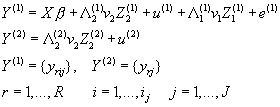(7.7)

The response superscript refers to the level at which the measurement exists, so that, for example, y 1ij, y2j refer respectively to the first measurement in the i-th level 1 unit in the j-th level 2 unit (say students and schools) and the second measurement taken at school level for the j-th school. Superscripts on the right hand side of (7.7) link to the appropriate response. Model (7.7) can also be incorporated into a SEM analogous to (7.6).
• P 180: 'Plewis (1985; Chapter 7)'
• P 183: Equation (10.9) should read:• P 183-184: Delete from 'and uijk
• P 184: 13 lines up change (10.10) to (10.9)
• P 190: equation (11.2) replace capital Y by lower case y.
• P 201: 'Browne et al. (2001b)'
• P 202: 'Goldstein et al. (2000a)'
• P 221: Equation (14.5) should read:• ADD to references: Leyland, A. (2001). Spatial Analysis. Multilevel Modelling of Health Statistics. In: A. Leyland and H. Goldstein. Multilevel Modelling of Health Statistics. Chichester, Wiley.
• Delete from references: Burdick et al.
• Appendix 2.4: replace steps 3 & 4 in the Gibbs sampling algorithm with the following:

Step 3:
Sample a new level 2 variance.
An issue arises here over the choice of a suitable diffuse prior distribution. A detailed discussion of this choice is given by Browne (1998) and we will here just mention two possibilities, a uniform prior as in the case of the fixed parameters and an inverse gamma prior. The former choice will tend often to produce positively biased estimates and the latter is commonly used. Rather than sampling the variance directly it is simpler to sample its inverse σu-2, referred to as the 'precision' which is assumed to have the gamma prior distribution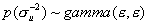.

We sample fromFor a uniform prior for σu2 we sample from a gamma distribution with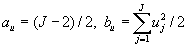.

Where there are p (>1) random coefficients we need to sample a new covariance matrixand corresponding to the two choices for a single variance are a set of uniform priors or an inverse Wishart prior. We now sample from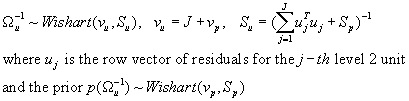A 'minimally informative' or 'maximally diffuse' choice for the prior would be to takeequal to the order ofandequal to a value chosen to be close to the final estimate multiplied by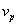; typically we can use the maximum likelihood or approximate maximum likelihood estimate forthat is also used for the MCMC starting values. Choosing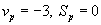is equivalent to choosing a uniform prior for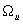Step 4
Sample a new level 1 variance.
This is similar to the procedure for a single level 2 variance. We sample fromFor a uniform prior we can sample from a gamma distribution with.
• Chapter 7: Eqn 7.8: Replace text about here with

Suppose we have a set of binary responses for a set of variables (level 1) measured on individuals (level 2). We can write a model analogous to (7.5) as(7.8)

where we now need to include at least an intercept in the fixed part and we can choose a logit or probit link function. The level 1 variances are effectively replaced by those from the independent binary variables. Extensions to higher levels follow straightforwardly (Goldstein and Browne, 2002b). For the probit link we can use a Gibbs sampler (see Appendix 4.3) where the lowest level variables are also thought of as latent, and generalisations to several levels and to structural equations follow similar lines to those.
• Appendix 2.2: Replace (below (2.2.4)) 'if we write …(2.2.7)' by:

If we write the l-level model as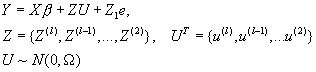(2.2.5)

then we can derive the following form for the composite residual estimator for levels 2 – l that is computationally convenient.(2.2.6)

with comparative covariance matrix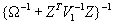Corresponding to (2.2.3) we have the following additional correction factor, to be subtracted from this covariance matrix, that takes into account the sampling variation of the fixed coefficients(2.2.7)